# Pressure Drop Calculation Models of Wellbore Fluid in Perforated Completion Horizontal Wells

Pressure Drop Calculation Models of Wellbore Fluid in Perforated Completion Horizontal Wells

Li HuaLu Yan Peng Xiaodong Lv Xindong Wang Laichao

CNOOC Ltd._Zhanjiang, Zhanjiang, Guangdong, China

Corresponding Author Email:
lihua8@cnooc.com.cn
Page:
65-72
|
DOI:
https://doi.org/10.18280/ijht.340110
|
Accepted:
|
Published:
31 March 2016
| Citation

OPEN ACCESS

Abstract:

Perforated completion is a main method of horizontal well completion. Based on the mass conservation equation, the momentum conservation equation and the variable mass flow in the horizontal wellbore, pressure drop calculation models of the wellbore fluid are established in perforated completion of horizontal wells. The results of calculation and analysis show that the frictional pressure drop, acceleration pressure drop and mixing pressure drop have different effects on total pressure drop of the wellbore fluid, and the frictional pressure drop plays a major role while the acceleration pressure drop and mixing pressure drop have little influence. The liquid viscosity, production and horizontal wellbore length also have different effects on various kinds of pressure drop. When liquid viscosity is smaller and the length of the horizontal wellbore is shorter, the effects of the acceleration pressure drop and mixing pressure drop cannot be neglected. The theoretical basis and the calculation model of the variable mass flow pressure drop of horizontal wellbore are provided.Pressure drop of wellbore fluid, Variable Mass Flow, Stratified flow, Perforated completion, Horizontal well.

Keywords:

Pressure drop of wellbore fluid, Variable Mass Flow, Stratified flow, Perforated completion, Horizontal well.

1. Introduction

In perforated completion of a horizontal well, due to variable mass flow of fluid in the horizontal wellbore, there is a drop in pressure along the horizontal wellbore, which will have an effect on fluid seepage and easily cause bottom water coning. Much research [1-23] has been done on pressure drop of horizontal wellbore. Scholars derived pressure drop model of horizontal wellbore, and obtained the variation law of pressure drop, as well as its impact on fluid flow. However this research has not considered the many forms of pressure drop which affect total pressure drop of horizontal wellbore systematically, without quantitatively analyzing the impact of all forms of pressure drop on total pressure drop, and considering the proportion of various forms of pressure drop to total pressure drop of a horizontal wellbore. Based on mass conservation equation and momentum conservation equation, this paper establishes a pressure drop model of perforated completion in a horizontal wellbore. It calculates and analyzes the impact of frictional pressure drop, acceleration pressure drop and mixing pressure drop on the total pressure drop in a horizontal wellbore. Furthermore, it analyzes the impact of viscosity, production, and length of a horizontal wellbore on the three kinds of pressure drop, as well as the impact boundary of acceleration pressure drop and mixing pressure drop on total pressure drop in a horizontal wellbore. It provides a theoretical basis and calculation model of pressure drop analysis of variable mass flow in perforated completion of a horizontal wellbore.

2. Modeling

It is assumed there is a gas/liquid two phase stratified flow in the horizontal wellbore as shown in Fig.1. The wellbore fluid is an isothermal flow, and there is no mass exchange in gas/liquid two phase. The pressure drop calculation model of horizontal wellbore is obtained from mass conservation equation and momentum conservation equation.

## figure_1a.png## figure_1b.png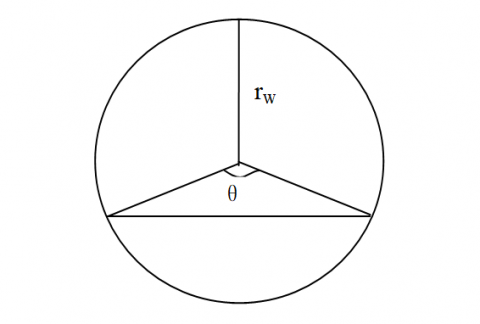Figure 1. Gas-liquid two phase flow

## figure_2.pngFigure 2. Balance of gas-liquid two phase flow

2.1 Mass conservation equation

$\left\{\begin{array}{l}{\Delta\left(\rho_{l} \cdot A_{l} \cdot U_{l}\right)=m_{l} \Delta x} \\ {\Delta\left(\rho_{g} \cdot A_{g} \cdot U_{g}\right)=m_{g} \Delta x}\end{array}\right.$(1)

In the equation $\rho_{l}$ is liquid density, kg/m3; $\rho_{g}$ is gas density, kg/m3; $A_{l}$ is liquid area, m2; $A_{g}$ is gas area, m2; $U_{l}$ is liquid velocity, m/s; $U_{g}$ is gas velocity, m/s; $m_{l}$ is liquid inflow per unit axis length, kg/(s×m); $m_{g}$ is gas inflow per unit axis length, kg/(s×m); $\Delta x$ is unit length, m.

Dividing both sides of the equation by$\Delta x$ yields:

$\left\{\begin{array}{l}{\frac{d\left(\rho_{l} \cdot A_{l} \cdot U_{l}\right)}{d x}=m_{l}} \\ {\frac{d\left(\rho_{g} \cdot A_{g} \cdot U_{g}\right)}{d x}=m_{g}}\end{array}\right.$(2)

Because:

$A_{l}=\pi r_{w}^{2} \cdot \alpha_{l}$(3)

$A_{g}=\pi r_{w}^{2} \cdot \alpha_{g}$(4)

$\alpha_{l}+\alpha_{g}=1$(5)

In the equation $r_{w}$ is wellbore radius, m; $\alpha_{l}$ is liquid holdup of wellbore fluid, decimals; $\alpha_{g}$ is gas holdup of wellbore fluid, decimals.

Integrating equations (3) and (4) into equation (2) yields:

$\left\{\begin{array}{l}{\frac{d\left(\rho_{l} \cdot U_{l} \cdot \alpha_{l}\right)}{d x}=\frac{m_{l}}{\pi r_{w}^{2}}} \\ {\frac{d\left(\rho_{g} \cdot U_{g} \cdot \alpha_{g}\right)}{d x}=\frac{m_{g}}{\pi r_{w}^{2}}}\end{array}\right.$(6)

In equation (6) :

$m_{l}=\frac{\ln \left(\frac{r_{e p}}{r_{p}}\right)-\sqrt{\left[\ln \left(\frac{r_{e p}}{r_{p}}\right)\right]^{2}-4 \frac{\rho_{l}\left(k_{r} k\right)^{2}}{\mu_{l}^{2}} \beta_{l}\left(\frac{1}{r_{e p}}-\frac{1}{r_{p}}\right)\left(p_{e p}-p_{p}\right)}}{\frac{k_{n} k}{\pi \mu_{l} L_{p}} \beta_{l}\left(\frac{1}{r_{e p}}-\frac{1}{r_{p}}\right)}$(7)

$m_{g}=\frac{\ln \left(\frac{r_{e p}}{r_{p}}\right)-\sqrt{\left[\ln \left(\frac{r_{e p}}{r_{p}}\right)\right]^{2}-4 \frac{\rho_{g}\left(k_{r g} k\right)^{2}}{\mu_{g}^{2} Z R T} \beta_{g}\left(\frac{1}{r_{e p}}-\frac{1}{r_{p}}\right)\left(p_{e p}^{2}-p_{p}^{2}\right)}}{\frac{k_{r g} k}{\pi \mu_{g} L_{p}} \beta_{g}\left(\frac{1}{r_{e p}}-\frac{1}{r_{p}}\right)}$(8)

In the equation, $r_{e p}$ is equivalent perforation radius, m; $r_{p}$ is inner perforation diameter, m; $k_{r l}$ is relative permeability of liquid; $k$ is formation permeability, μm2; $\mu_{l}$ is liquid viscosity, mPa·s; $\beta_{l}$ is velocity factor of liquid; $P_{e p}$ is corresponding pressure of equivalent perforation radius, Pa; $P_{e p}$ is corresponding pressure of inner perforation diameter, Pa; $k_{r g}$$k_{r g} is relative permeability of gas; \mu_{g} is gas viscosity, mPa·s; Z is gas compression coefficient; R is coefficient of gas state equation; T is wellbore temperature, K; \beta_{g} is velocity factor of gas. 2.2 Momentum conservation equation (1) Momentum conservation equation of liquid \Delta\left(\rho_{l} \cdot A_{l} \cdot U_{l}^{2}\right) \cdot \Delta t=-\Delta P_{a c l} \cdot A_{l} \cdot \Delta t-\Delta P_{m i n l} \cdot A_{l} \cdot \Delta t$$-\tau_{l} S_{l} \Delta x \cdot \Delta t+\tau_{\mathrm{lg}} S_{\mathrm{lg}} \Delta x \cdot \Delta t$(9)

In the equation $P_{a c c l}$ is acceleration pressure drop of liquid, Pa; $P_{m i x i}$ is mixing pressure drop of liquid, Pa; $\tau_{l}$ is shear stress of liquid and wall, Pa; $\tau_{\mathrm{lg}}$ is shear stress of gas/liquid interface, Pa; $S_{l}$ is liquid boundary length of flow cross section, m; $S_{\lg }$ is gas/liquid interface length, m; $\Delta t$ is time of liquid flow through unit length, S.

(2) Momentum conservation equation of gas

$\Delta\left(\rho_{g} \cdot A_{g} \cdot U_{g}^{2}\right) \cdot \Delta t=-\Delta P_{a c c g} \cdot A_{g} \cdot \Delta t-\Delta P_{m i x g} \cdot A_{g} \cdot \Delta t$$-\tau_{g} S_{g} \Delta x \cdot \Delta t-\tau_{\lg } S_{\mathrm{lg}} \Delta x \cdot \Delta t(10) In the equation P_{a c c g} is acceleration pressure drop of gas, Pa; P_{\text {mixg}} is mixing pressure drop of gas, Pa; \tau_{g} is shear stress of gas and wall, Pa; S_{g} is gas boundary length of flow cross section, m. (3) Momentum conservation equation of fluid -\Delta P \cdot A \cdot \Delta t=-\Delta P_{a c c l} \cdot A_{l} \cdot \Delta t-\Delta P_{m i x l} \cdot A_{l} \cdot \Delta t-\tau_{l} S_{l} \Delta x \cdot \Delta+\tau_{\lg } S_{\lg } \Delta x \cdot \Delta -\Delta P_{a c c g} \cdot A_{g} \cdot \Delta t-\Delta P_{m i n g} \cdot A_{g} \cdot \Delta t-\tau_{g} S_{g} \Delta x \cdot \Delta t-\tau_{\lg } S_{\mathrm{lg}} \Delta x \cdot \Delta t (11) In the equation P is total pressure drop of fluid, Pa. So: \Delta\left(\rho_{i} \cdot A_{l} \cdot U_{l}^{2}\right) \cdot \Delta t=-\Delta P \cdot A \cdot \Delta t+\Delta P_{a c c g} \cdot A_{g} \cdot \Delta t$$+\Delta P_{m n g g} \cdot A_{g} \cdot \Delta t+\tau_{g} S_{g} \Delta x \cdot \Delta t+\tau_{\mathrm{lg}} S_{\mathrm{lg}} \Delta x \cdot \Delta t$(12)

$\Delta\left(\rho_{g} \cdot A_{g} \cdot U_{g}^{2}\right) \cdot \Delta t=-\Delta P \cdot A \cdot \Delta t+\Delta P_{a c l} \cdot A_{l} \cdot \Delta t$$+\Delta P_{m i n l} \cdot A_{l} \cdot \Delta t+\tau_{l} S_{l} \Delta x \cdot \Delta t-\tau_{\mathrm{lg}} S_{\mathrm{lg}} \Delta x \cdot \Delta t(13) Thereinto: \Delta P_{a c c l}=\frac{3 \rho_{l} U_{l i}^{2} \cdot m_{l p}+3 U_{l p} \cdot \frac{m_{l p}^{2}}{\pi r_{w}^{2} \cdot \alpha_{l}}+\frac{m_{l p}^{3}}{\rho_{l}^{2}\left(\pi r_{w}^{2} \cdot \alpha_{l}\right)^{2}}-m_{l p} \frac{1}{\rho_{l}}\left(\frac{m_{l p}}{\pi r_{p}^{2} \cdot \alpha_{l}}\right)^{2}}{2 \rho_{l} \pi r_{w}^{2} U_{l i} \cdot \alpha_{l}+m_{l p}} (14) \Delta P_{a c c g}=\frac{3 \rho_{g} U_{g i}^{2} \cdot m_{g p}+3 U_{g i} \cdot \frac{m_{g p}^{2}}{\pi r_{w}^{2} \cdot \alpha_{g}}+\frac{m_{g p}^{3}}{\rho_{g}^{2}\left(\pi r_{w}^{2} \cdot \alpha_{g}\right)^{2}}-m_{g p} \frac{1}{\rho_{g}}\left(\frac{m_{g p}}{\pi r_{p}^{2} \cdot \alpha_{g}}\right)^{2}}{2 \rho_{g} \pi r_{w}^{2} U_{g i} \cdot \alpha_{g}+m_{g p}} (15) \Delta P_{m i x l}=\frac{3 \cdot m_{l p}}{\pi r_{w}^{2}} \cdot \frac{U_{l i}}{\alpha_{l}}+\frac{3 m_{l p}^{2}}{2 \rho_{l}\left(\pi r_{w}^{2}\right)^{2}} \cdot \frac{1}{\alpha_{l}^{2}}$$-\frac{3 \rho_{l} U_{l i}^{2} \cdot m_{l p}+3 U_{h} \cdot \frac{m_{l p}^{2}}{\pi r_{w}^{2} \cdot \alpha_{l}}+\frac{m_{p}}{\rho_{l}^{2}\left(\pi r_{w}^{2} \cdot \alpha_{l}\right)^{2}}-m_{l p} \frac{1}{\rho_{l}}\left(\frac{m_{l p}}{\pi r_{p}^{2} \cdot \alpha_{l}}\right)^{2}}{2 \rho_{l} \pi r_{w}^{2} U_{l i} \cdot \alpha_{l}+m_{l p}}$ (16)

$\Delta P_{\text {mixg}}=\frac{3 \cdot m_{g p}}{\pi r_{w}^{2}} \cdot \frac{U_{g i}}{\alpha_{g}}+\frac{3 m_{g p}^{2}}{2\left(\pi r_{w}^{2}\right)^{2}} \cdot \frac{1}{\rho_{g} \cdot \alpha_{g}^{2}}$$-\frac{3 \rho_{g} U_{g i}^{2} \cdot m_{g p}+3 U_{g i} \cdot \frac{m_{g p}^{2}}{\pi r_{w}^{2} \cdot \alpha_{g}}+\frac{m_{g p}^{3}}{\rho_{g}^{2}\left(\pi r_{w}^{2} \cdot \alpha_{g}\right)^{2}}-m_{g p} \frac{1}{\rho_{g}}\left(\frac{m_{g p}}{\pi r_{p}^{2} \cdot \alpha_{g}}\right)^{2}}{2 \rho_{g} \pi r_{w}^{2} U_{g i} \cdot \alpha_{g}+m_{g p}}$ (17)

From Fig.1 yields:

$S_{l}=r_{w} \cdot \theta$(18)

$S_{g}=r_{w} \cdot(2 \pi-\theta)$(19)

$S_{\mathrm{lg}}=2 \cdot r_{w} \cdot \sin \left(\frac{\theta}{2}\right)$(20)

Dividing both sides of equation (12) by$\Delta t \cdot \Delta x$yields:

$\frac{d\left(\rho_{l} \cdot A_{l} \cdot U_{l}^{2}\right)}{d x}=-\frac{d P}{d x} \cdot A+\frac{d P_{a c g}}{d x} \cdot A_{g}+\frac{d P_{m i x g}}{d x} \cdot A_{g}+\tau_{g} S_{g}+\tau_{1 g} S_{\mathrm{lg}}$(21)

Integrating equations (3) and (4) into equation (21)yields momentum conservation equation of liquid:

$\frac{d\left(\rho_{l} \cdot U_{l}^{2} \cdot \alpha_{l}\right)}{d x}=-\frac{d P}{d x}+\frac{d P_{a c c g}}{d x} \cdot \alpha_{g}+\frac{d P_{m i r g}}{d x} \cdot \alpha_{g}+\frac{\tau_{g}}{\pi r_{w}^{2}} S_{g}+\frac{\tau_{\mathrm{lg}}}{\pi r_{w}^{2}} S_{\mathrm{lg}}$(22)

The momentum conservation equation of gas is similarly obtained:

$\frac{d\left(\rho_{g} \cdot U_{g}^{2} \cdot \alpha_{g}\right)}{d x}=-\frac{d P}{d x}+\frac{d P_{a c l}}{d x} \cdot \alpha_{l}+\frac{d P_{m i x l}}{d x} \cdot \alpha_{l}+\frac{\tau_{l}}{\pi r_{w}^{2}} S_{l}-\frac{\tau_{\lg }}{\pi r_{w}^{2}} S_{\mathrm{lg}}$(23)

2.3 Calculation model of horizontal wellbore pressure drop

Combining mass conservation Eq(6) and momentum conservation Eq (22), (23) yields:

\left\{ \begin{align} & \frac{d\left( {{\rho }_{l}}\cdot {{U}_{l}}\cdot {{\alpha }_{l}} \right)}{dx}=\frac{{{m}_{l}}}{\pi {{r}_{w}}^{2}} \\ & \frac{d\left( {{\rho }_{g}}\cdot {{U}_{g}}\cdot {{\alpha }_{g}} \right)}{dx}=\frac{{{m}_{g}}}{\pi {{r}_{w}}^{2}} \\ & \frac{d\left( {{\rho }_{l}}\cdot {{U}_{l}}^{2}\cdot {{\alpha }_{l}} \right)}{dx}=-\frac{dP}{dx}+\frac{d{{P}_{accg}}}{dx}\cdot {{\alpha }_{g}}+\frac{d{{P}_{mixg}}}{dx}\cdot {{\alpha }_{g}}+\frac{{{\tau }_{g}}}{\pi {{r}_{w}}^{2}}{{S}_{g}}+\frac{{{\tau }_{\lg }}}{\pi {{r}_{w}}^{2}}{{S}_{\lg }} \\ & \frac{d\left( {{\rho }_{g}}\cdot {{U}_{g}}^{2}\cdot {{\alpha }_{g}} \right)}{dx}=-\frac{dP}{dx}+\frac{d{{P}_{accl}}}{dx}\cdot {{\alpha }_{l}}+\frac{d{{P}_{mixl}}}{dx}\cdot {{\alpha }_{l}}+\frac{{{\tau }_{l}}}{\pi {{r}_{w}}^{2}}{{S}_{l}}-\frac{{{\tau }_{\lg }}}{\pi {{r}_{w}}^{2}}{{S}_{\lg }} \\ \end{align} \right. (24)

Eq(24) can be resolved by the fourth order Runge-Kutta method.

3. Result Analysis

Basic data of wellbore pressure drop analysis of perforated completion model in horizontal well is shown in Tab. 1.

Table 1. Based data of pressure drop analysis model in horizontal well

 Equivalent drainage radius(m) 374 Reservoir thickness (m) 20 Horizontal wellbore length(m) 419 Effective wellbore diameter (m) 0.1594 Effective perforation depth(m) 0.55 Effective perforation radius (m) 0.006 Permeability(μm2) 0.06 Toe end pressure of horizontal wellbore (MPa) 20 Initial perforation density (hole/m) 20 Total production(m3/d) 7560 Liquid density(kg/m3) 900 Liquid viscosity(mPa·s) 50 Coefficient of gas state equation (J/kg/K) 200 Gas viscosity(mPa·s) 0.02 Relative liquid permeability 0.2 Wellbore temperature(K) 380 Non-Darcy flow coefficient 2.33 Relative gas permeability 0.8 Friction coefficient 0.316 Index of friction coefficient 0.19 Initial liquid holdup 0.04 Gas compressibility 1

3.1 Component analysis of total pressure drop in horizontal wellbore

Pressure drop of fluid in horizontal wellbore consists of three parts: (1) frictional pressure drop caused by gas/liquid interaction as well as friction of gas/liquid and wellbore wall; (2) inflowing fluid through perforation mixes with fluid in horizontal wellbore, and fluid redistribution causes mixed pressure drop; (3) radial flow and liquid holdup change causes flow rate change of gas and liquid, which contributes to acceleration pressure drop. Calculation result is shown in Fig. 3.

## figure_3.png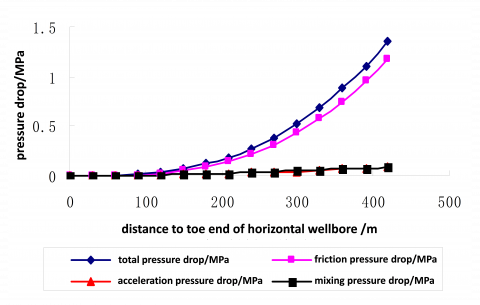Figure 3. Various types of pressure drop curve in horizontal wellbore

Fig. 3 shows that when fluid flows through toe end to heel end of the horizontal wellbore, the total pressure drop of the horizontal wellbore is 1.36MPa. Friction pressure drop accounts for more than 85%, while the percentage of acceleration pressure drop and mixed pressure drop is relatively small.

3.2 Impact of viscosity on horizontal wellbore pressure drop

While other conditions remain unchanged, with liquid viscosity of 50mPa·s, 100mPa·s, 500mPa·s, 1000mPa·s, 5000mPa·s and 10000mPa·s, the total pressure drop curve is shown in Fig. 4, friction pressure drop curve is shown in Fig. 5, acceleration pressure drop curve is shown in Fig. 6, and mixed pressure drop curve of horizontal wellbore is shown in Fig. 7.

## figure_4.png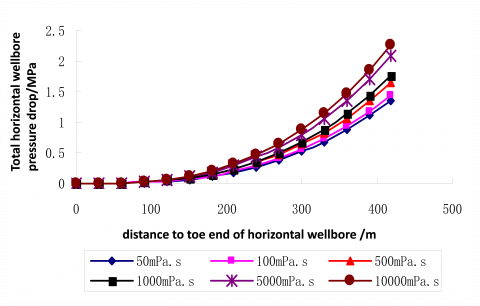Figure 4. General pressure drop curve in horizontal wellbore

## figure_5.png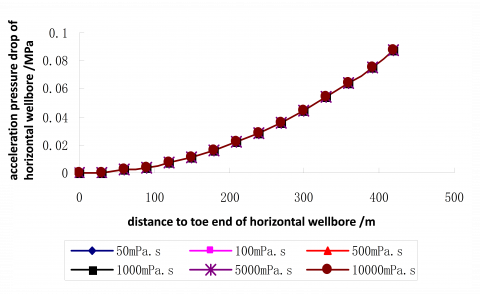Figure 5. Frictional pressure drop curve in horizontal wellbore

Fig. 4 and Fig. 5 show that when liquid viscosity increases, total pressure drop and friction pressure drop increase gradually. The impact of liquid viscosity on total pressure drop and friction pressure drop in horizontal wellbore is obvious.

## figure_6.pngFigure 6. Acceleration pressure drop curve in horizontal wellbore

## figure_7.png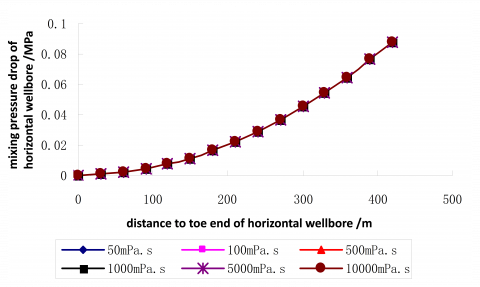Figure 7. Mixing pressure drop curve in horizontal wellbore

Fig. 6 and Fig. 7 show that liquid viscosity has less influence on accelerating pressure drop and mixing pressure drop in horizontal wellbore.

3.3 Impact of production on horizontal wellbore pressure drop

While other conditions remain unchanged, with production of 100m3/d, 500m3/d, 1000m3/d, 5000m3/d, 8000m3/d and 10000m3/d, the total pressure drop curve is shown in Fig. 8, friction pressure drop curve is shown in Fig. 9, acceleration pressure drop curve is shown in Fig. 10, and mixing pressure drop curve of horizontal wellbore is shown in Fig. 11.

## figure_8.png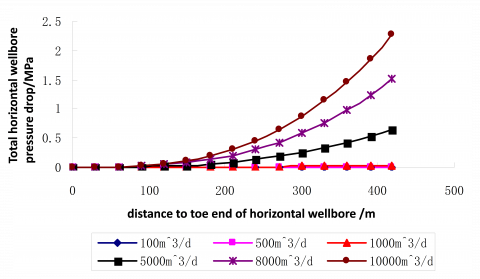Figure 8. Total pressure drop curve in horizontal wellbore

## figure_9.pngFigure 9. Friction pressure drop curve in horizontal wellbore

Fig. 8 and Fig. 9 show that production has a large effect on total pressure drop and friction pressure drop in the horizontal wellbore. When production increases, total pressure drop and friction pressure drop in the horizontal wellbore increase gradually.

## figure_10.pngFigure 10. Acceleration pressure drop curve in horizontal wellbore

## figure_11.png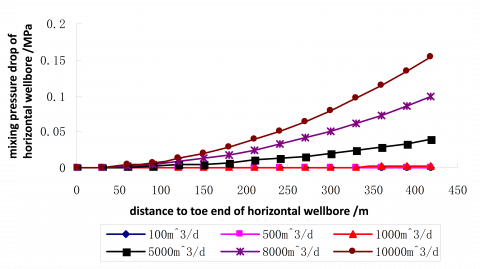Figure 11. Mixing pressure drop curve in horizontal wellbore

Fig. 10 and Fig. 11 show that production also has a large effect on acceleration pressure drop and mixing pressure drop in the horizontal wellbore. When production increases, acceleration pressure drop and mixing pressure drop in the horizontal wellbore increase gradually.

3.4 Impact of horizontal section length on horizontal wellbore pressure drop

While other conditions remain unchanged, with horizontal section length of 200m, 500m, 800m, 1000m, 1500m and 2000m, the total pressure drop curve is shown in Fig. 12, friction pressure drop curve is shown in Fig. 13, acceleration pressure drop curve is shown in Fig. 14, and mixing pressure drop curve of horizontal wellbore is shown in Fig. 15.

## figure_12.png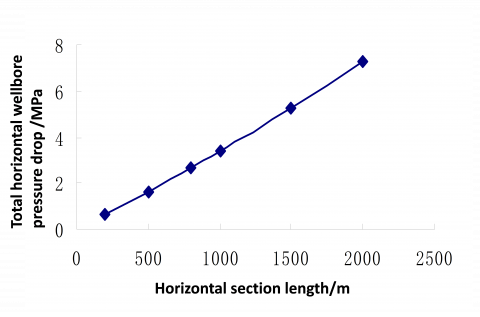Figure 12. General pressure drop curve in horizontal wellbore

## figure_13.png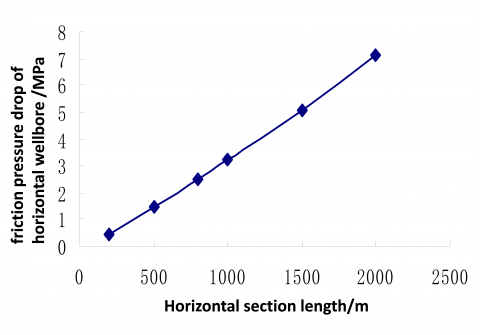Figure 13. Friction pressure drop curve in horizontal wellbore

Fig. 12 and Fig. 13 show that the horizontal section length has a large effect on total pressure drop and friction pressure drop in the horizontal wellbore. When the horizontal section length increases, total pressure drop and friction pressure drop in the horizontal wellbore increase gradually.

## figure_14.png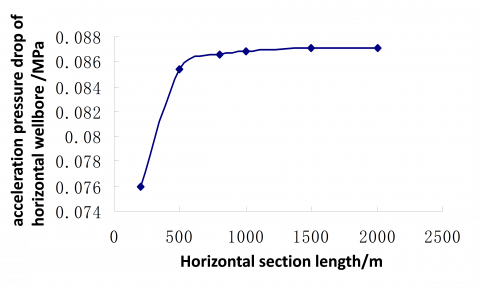Figure 14. Acceleration pressure drop curve in horizontal wellbore

## figure_15.png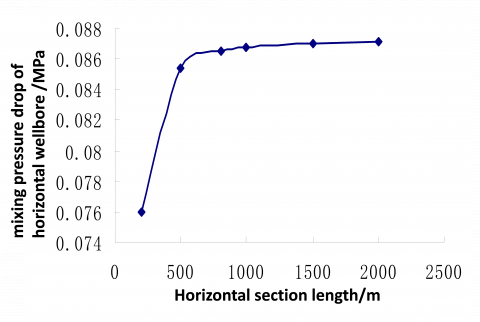Figure 15. Mixing pressure drop curve in horizontal wellbore

Fig. 14 and Fig. 15 show that the horizontal section length has less effect on acceleration pressure drop and mixing pressure drop in horizontal wellbore. When the horizontal section length increases, acceleration pressure drop and mixing pressure drop in the horizontal wellbore increase gradually, but increasing amplitude decreases.

3.5 Impact boundary of acceleration pressure drop on total pressure drop in horizontal wellbore

When acceleration pressure drop is less than 5% of the total wellbore pressure drop, the impact of acceleration pressure drop on the total horizontal wellbore pressure drop is negligible; but when acceleration pressure drop is more than 5% of the total wellbore pressure drop, the impact of acceleration pressure drop on the total horizontal wellbore pressure drop must be considered. From the above analysis, the main factors that affect total horizontal wellbore pressure drop are liquid viscosity, production and horizontal section length. Friction pressure drop is mainly influenced by liquid viscosity, production and horizontal section length. Acceleration pressure drop and mixing pressure drop are mainly influenced by the horizontal section length. With a horizontal well production of 7560m3/d, the relation curve indicating the impact boundary of acceleration pressure drop on total horizontal wellbore pressure drop is shown in Fig 16, where μ is liquid viscosity, mPa·s. To liquid viscosity and horizontal section length, when eligible points are at the bottom-left of the curve, the impact of accelerating pressure drop on the total horizontal wellbore pressure drop is negligible. When they are at the top-right of the curve, the impact of acceleration pressure drop on total horizontal wellbore pressure drop must be considered.

Similarly the impact boundary of mixing pressure drop on total horizontal wellbore pressure drop is shown in Fig. 17.

## figure_16.png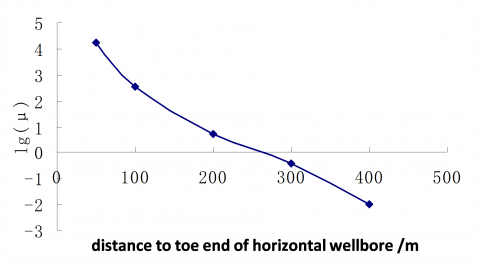Figure 16. Boundary curve of accelerating pressure drop impact on general pressure drop

## figure_17.pngFigure 17. Boundary curve of mixing pressure drop impact on total pressure drop

4. Conclusions

(1) Considering variable mass flow of fluid in the horizontal wellbore, based on mass conservation equation and momentum conservation equation, the pressure drop model of perforated completion in horizontal wellbore is established. In order to analyze, the total horizontal wellbore pressure drop is divided into friction pressure drop, acceleration pressure drop and mixing pressure drop. This provides a theoretical basis and calculation model for pressure drop analysis of variable mass flow in horizontal wellbore.

(2) Normally, friction pressure drop plays a leading role in total horizontal wellbore pressure drop. Accelerating pressure drop and mixing pressure drop has less effect on the total horizontal wellbore pressure drop.

(3) Liquid viscosity mainly affects the total horizontal wellbore pressure drop by friction pressure drop. When liquid viscosity is of a higher degree, friction pressure drop and total pressure drop of the horizontal wellbore is larger. Horizontal well production has an obvious effect on total horizontal wellbore pressure drop, friction pressure drop, acceleration pressure drop, and mixing pressure drop. With an increase in horizontal well production, many types of pressure drop in the horizontal wellbore increases. Horizontal section length mainly affects the total horizontal wellbore pressure drop by effect of horizontal wellbore friction pressure drop, and it has less effect on accelerating pressure drop and mixing pressure drop.

(4) The impact boundary of acceleration pressure drop and mixing pressure drop on total horizontal wellbore pressure drop has been studied, and the boundary curve plate has been obtained.

References

 S. T. Zhou and Q. Zhang, “An analytic model of horizontal section pressure drop,” Petroleum Exploration and Development, vol. 24, no. 3, pp.49-52, 1997. DOI: 10.3321/j.issn: 1000-0747.1997.03.012.

 Q. Zhang, S. T. Zhou, N. Wu and M. Z. Li, “Gas-liquid two phases variable mass flowing low in horizontal well,” China University of Petroleum Journal, vol. 26, no. 6, pp. 20-26, 2002. DOI: 10.3321/j.issn: 1000-5870.2002.06.013.

 B. Z. Li, W. J. Song and S. H. Ji, “Pressure distribution behavior of horizontal section in horizontal well,” Acta Petrolei Sinica, vol. 24, no. 2, pp. 97-100, 2003. DOI: 10.7623/syxb200302021.

 F. J. Sun, Y. D. Xu, L. S. Cheng and X. S. Li, “A calculation model of pressure drop for stratified flow in horizontal well,” China Offshore Oil, vol. 16, no. 2, pp. 98-100 2004. DOI: 10.3969/j.issn.1673-1506.2004.02.005.

 X. P. Li, L. H. Zhang and Y. Li, “Variation laws of pressure and production rate in horizontal well,” Journal of Hydrodynamics, vol. 20, no. 4, pp. 492-496, 2005. DOI: 10.3969/j.issn.1000-4874.2005.04.013.

 X. Q. Wang, Z. M. Wang and J. G. Wei, “Variable mass flowing low in horizontal well under wellbore and reservoir coupling,” Journal of Hydrodynamics, vol. 20, no. (4), pp. 326-331, 2005.

 D. C. Chen, H. R. Hai, Z. P. Zhang and X. C. Huang, “Research on inflow performance relationship of oil - gas -water three phases for horizontal wells,” Petroleum Geology and Recovery Efficiency, vol. 13, no. 3, pp. 50-52, 2006. DOI: 10.3969/j.issn.1009-9603.2006.03.015.

 Q. Wang, H. Q. Liu and L. Meng, “Influencing factors to horizontal well productivity considering wellbore pressure drop,” Special Oil & Gas Reservoirs, vol. 16, no. 5, pp. 48-57, 2009. DOI: 10.3969/j.issn.1006-6535.2009.05.013.

 X. D. Peng, X. G. Wang, et al, “A new method for deliverability evaluation of offshore gas reservoir with high temperature and pressure,” Environmental and earth Sciences Research Journal, vol. 2, no. 1, pp. 1-6, 2015. DOI: 10.18280/EESRJ.020101.

 M. Li and Q. Sang, “The research of the method of identifying low resistivity reservoir in Zhusan depression of the pearl river mouth basin,” Environmental and Earth Sciences Research Journal, vol. 1, no. 1, pp. 11-16, 2014. DOI: 10.18280/eesrj.010103.

 R.M.S.M. Schulkes and O. H. Utvik, “Pressure drop in a perforated pipe with radial inflow: single-phase flow,” Spe Journal, vol. 3, no. 1, pp. 77-85, 1998. DOI: 10.2118/38448-PA.

 M. Bourenane, D. Tiab and R. Recham, “Optimization of perforated completions for horizontal wells in a high-permeability, thin oil zone. Case Study: Hassi R’mel Oil Rim, Algeria,” Presented at Canadian International Petroleum Conference, 2004. DOI: 10.2118/2004-123.

 J. Ansah, M. Proett and M. Soliman, “Advances in well completion design: A new 3D finite-element wellbore inflow model for optimizing performance of perforated completions,” presented at International Symposium and Exhibition on Formation Damage Control Conference, Feb. 2002. DOI: 10.2118/73760-MS.

 D. Denney, “Intelligent wells: Horizontal-well simulation for thin oil rims in the Niger Delta - A case study,” Journal of Neuroscience Research, vol. 94, no. 1, pp. 135-135, 2016. DOI: 10.2118/1110-0074-JPT.

 K. S. Chan, R. Masoudi, H. Karkooti, R. Shaedin and M. B. Othman, “Smart horizontal well drilling and completion for thin oil-rim reservoirs in Malaysia,” Journal of Petroleum Technology, pp. 103-107, 2016.

 E. Sarvaramini and D. Garagash, “On the production analysis of a multi-fractured horizontal well,” in New Frontiers in Oil and Gas Exploration, Springer Press, 2016.

 K. Bybee, “Improved horizontal-well stimulations in the Bakken Formation”, Journal of Petroleum Technology, vol. 56, no. 11, pp. 49-50, 2015. DOI: 10.2118/1104-0049-JPT.

 A.R.A. Bajalan, “Planning UBD in horizontal well to minimize formation damage,” Journal of Petroleum & Environmental Engineering, vol. 06, no. 2, 2015. DOI: 10.4172/2157-7463.1000212.

 Y. Mahboub, M. Otmanine and B. Salhi, “Modeling and optimization of horizontal well completions located in gas injection patterns of Hassi Messaoud Oilfield,” presented at International Petroleum Technology Conference, 2015. DOI: 10.2523/IPTC-18553-MS.

 A. Briner, A. Moiseenkov, S. Nadezhdin, O. A. Zeidi and T. Batmaz, “Successfully planning horizontal well completions using advanced workflows in a tight gas reservoir in the Sultanate of Oman,” presented at SPE Production and Operations Symposium, 2015. DOI: 10.2118/173611-MS.

 McSpadden, A. R. and Trevisan, R, “Friction load redistribution for robust and cost efficient design of extended reach and horizontal well completions,” presented at IADC/SPE Drilling Conference and Exhibition, 2016. DOI: 10.2118/178905-MS.

 S. Nadezhdin, M. El Gihani, A. Al Alqam, A. Briner and O. Harrasi, “Microseismic monitoring reveals hydraulic fracture development in clustered horizontal well completed in high-temperature tight gas reservoir, Sultanate of Oman,” presented at SPE Oil & Gas India Conference and Exhibition, 2015. DOI: 10.2118/178130-MS.

 H. Dandan, G. Rui, H. Songhao, W. Yuanbing and Z. Zhaowu, “Integrated management and application of horizontal well water flooding technology in a large-scale complicated carbonate oilfield containing high permeability streaks,” presented at SPE/IATMI Asia Pacific Oil & Gas Conference and Exhibition, DOI: 10.2118/176110-MS.

 Z. M. Wang, J. Xu and X. Q. Wang, “Study on variable density perforating model of two-phase flow in horizontal wells,” University of Petroleum Journal, vol. 29, no. 3, pp. 36-40, 2005. DOI: 10.3321/j.issn: 1000-5870.2005.03.015.